# 向量运算

### 5.2 符号约定

• 标量，用斜体的小谢罗马或者希腊字母表示，如abθ
• 向量，用小写黑粗体字母表示，如abu
• 矩阵，用大写黑粗体字母表示，如ABR

### 5.3 零向量

n维向量集合的加性单位元就是n维“零向量”。(如[0 0 0 0 ···· 0])

### 5.4 负向量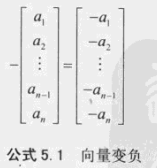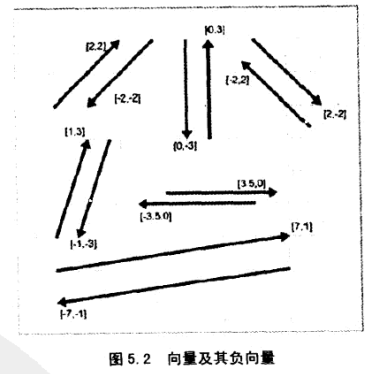### 5.5 向量大小(长度或模)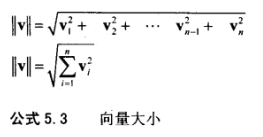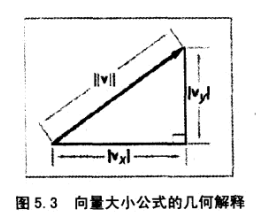### 5.6 标量与向量的乘法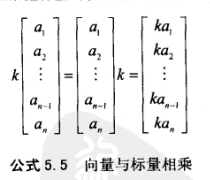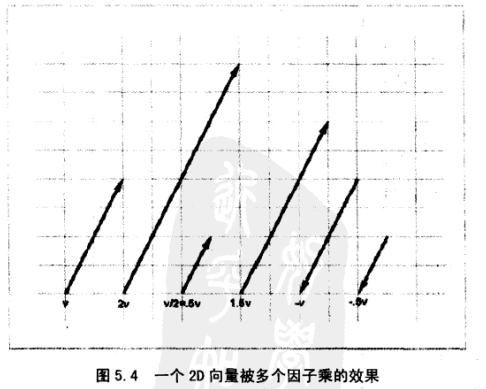### 5.7 标准化向量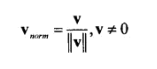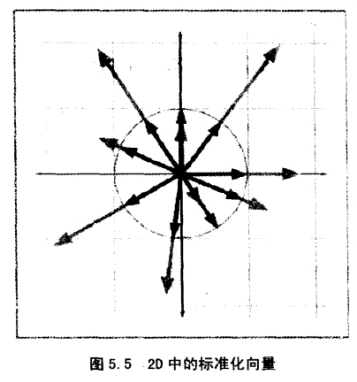### 5.8 向量的加法和减法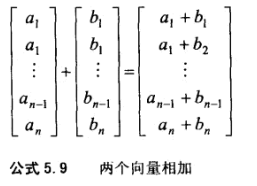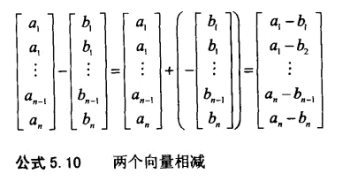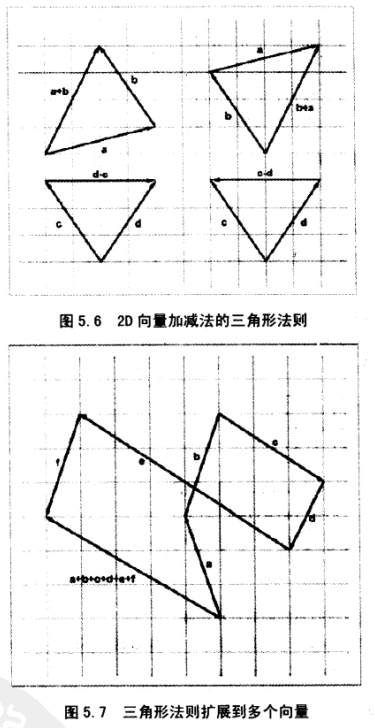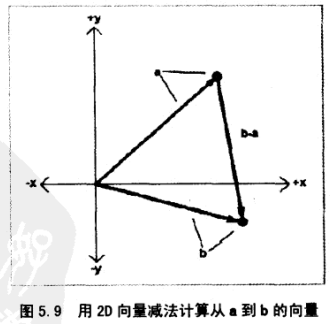### 5.9 距离公式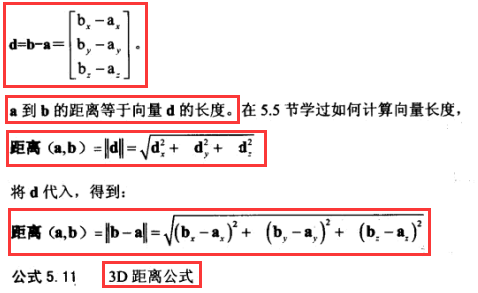### 5.10 向量点乘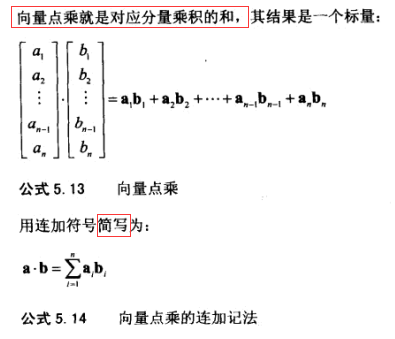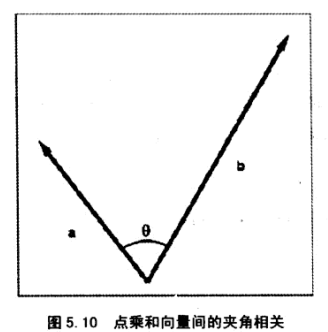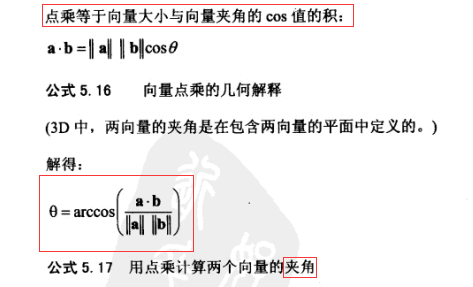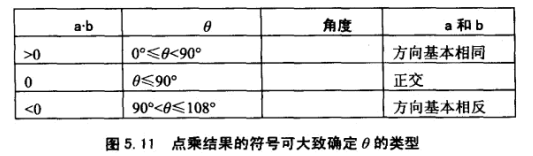### 5.12 线性代数公式

abs表示绝对值，mod表示模，sqrt表示开根号。

a+b=b+a 向量加法的交换律
a-b=a+(-b) 向量减法的定义
(a+b)+c a+(b+c)
s(ta)=(st)a 标量乘法的结合律
k(a+b)=ka+kb 标量乘法对向量加法的分配律
mod(ka)=abs(k)*mod(a) 向量乘以标量相当于标量的绝对值为因子缩放向量
mod(a)>=0 向量的大小非负
mod(a)^2+mod(b)^2=mod(a+b)^2 勾股定理
mod(a)+mod(b)>=mod(a+b) 向量加法的三角形法则(三角形两边之和大于或等于第三边)
a·b=b·a 点乘的交换律
mod(a)=sqrt(a*a) 用点乘定义向量大小
k(a·b)=(ka)·b=a·(kb) 标量乘法对点乘的结合律
a·(b+c)=a·b+a·c 点乘对向量加减法的分配律
axa=0 任意向量与自身的叉乘等于零向量
axb=-(bxa) 叉乘逆交换律
axb=(-a)x(-b) 叉乘的操作数同时变负得到相同的结果
k(axb)=(ka)xb=ax(kb) 标量乘法对叉乘的结合律
ax(b+c)=axb+axc 叉乘对向量加法的分配律
a·(axb)=0 向量与另一个向量叉乘再点乘该向量本身等于零©️2019 CSDN 皮肤主题: 大白 设计师: CSDN官方博客